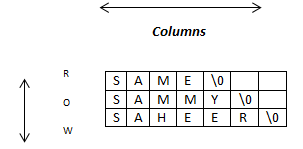• Arrays of string can be represented using the two dimension character array.
• An array having two subscripts is known as two-dimension array.
• Two-dimensional array is a collection of rows and columns so it can be represented for arrays of string easily.
• It can be represented as a series or collection of one-dimension character array. It allows storing data in tabular format.
• Individual characters can be represented by Vij where ‘i’ value indicates the row number and ‘j’ value indicated the column number.
##### Declaration and Initialization of Two-Dimension Array:
• We can declare two-dimension character array using the following syntax:
``char str-var-name [row size] [column size] ;``
• Here, <row size> and <column size> specifies total number of rows and columns respectively.
• The following example declares a two-dimensional string array with three strings.
``char names = { “SAME”, “SAMMY” , “SAHEER” };``
• Memory representation of string names can be given as follow• Here intersection of rows and columns creates a cell and each cell location can be identified by using its row index and column index.
• For example, the location of the first character will be 0th row and 0th column ({0, 0}), for second element 0th row and 1st column ( { 0, 1 } ), 1st row and 0th column for thirst element ( { 1, 0 } ) and 1st row and 1st column for fourth element ( { 1, 1 } ) and so on.
##### AccessingTwo-Dimension Array Elements:
• We can access each character array elements by using its row index as well as column index within square brackets along with array variable name.
• For example, to access the first string of the array names, you can use names , for second string names , and so on.
###### Dynamic initialization:
• We can also initialize array of string at run time. It is referred to as dynamically initialization.
• The following example uses for loop to initialize names two dimension array from user at run time.
``````for ( i=0 ; i < 2 ;  i++ )  //   iterates over rows
{
printf(“\n Enter Names[%d]:”, i + 1 ) ;
scanf( “ %s “ , &names [i] ) ;
}``````
• The following program demonstrates reading four string and displaying on the screen.
``````/* Program to read and display four strings. */

#include "stdio.h"

void main(void)
{
char names;    /* this declares stringarray gf 3 */
int i

clrscr();

/* read data from the keyboard  */

for ( i=0 ; i < 3 ;  i++ )  //   iterates over rows
{
printf(“\n Enter x[%d]:”, i + 1 ) ;
scanf( “%s“ , &names[i] ) ;
}

/* display data on the screen  */

for ( i=0 ; i < 3 ;  i++ )  //   iterates over rows
{
printf(“\n %s”, names[i] ) ;
}

}``````